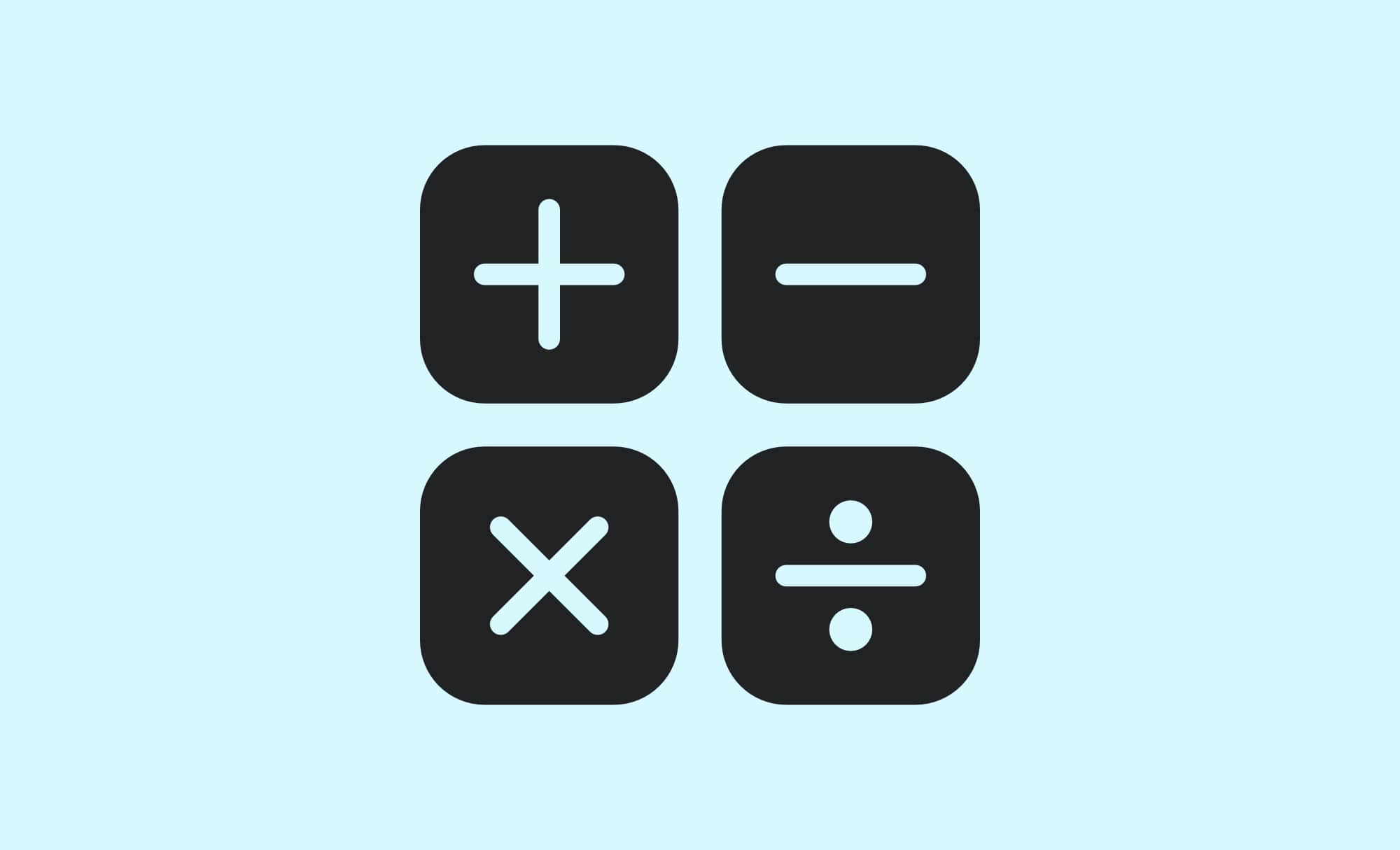# Maths74

GCSE Maths (Mathematics) learning topics and material for students in Year 7 to Year 11.

Check out the latest posts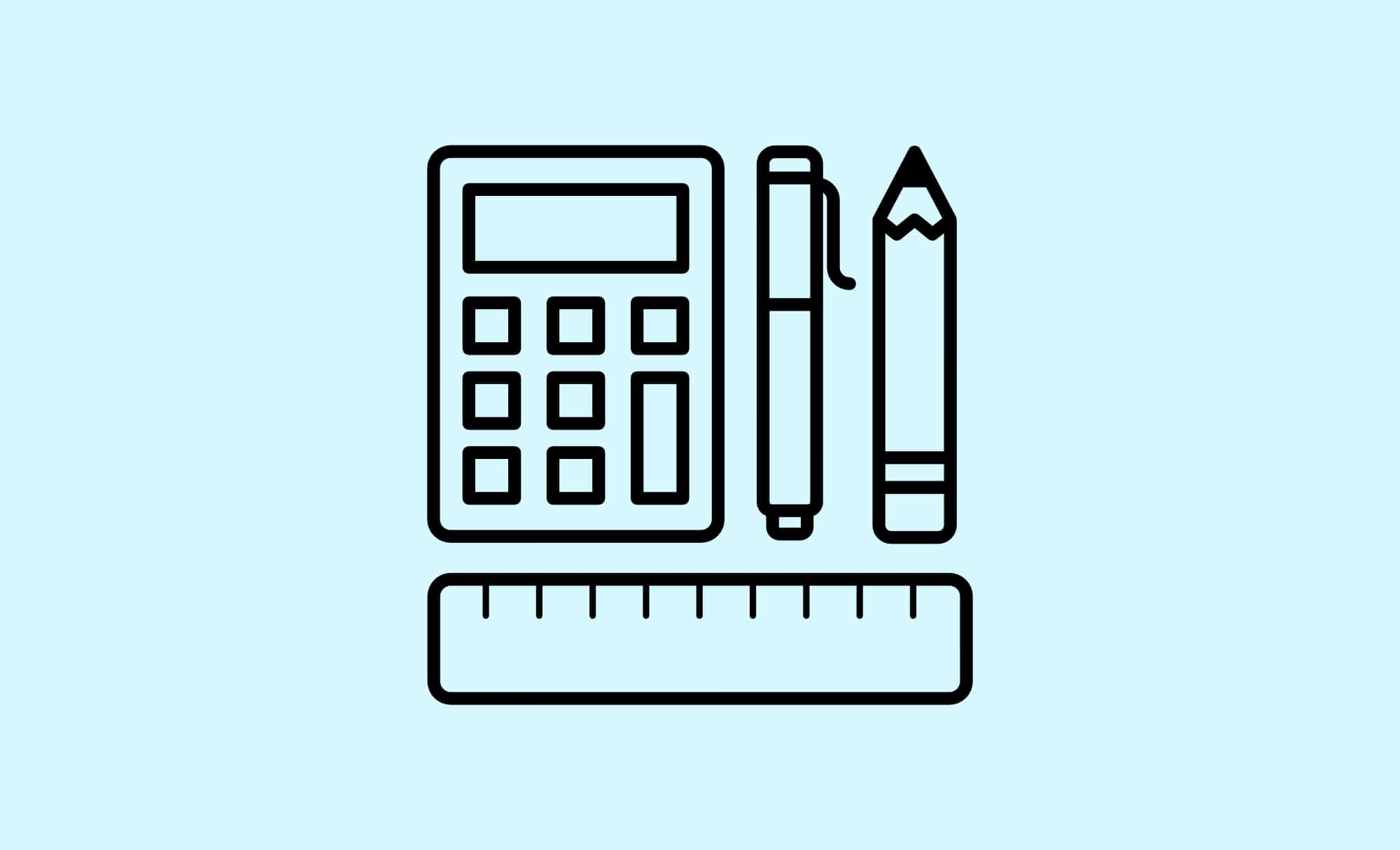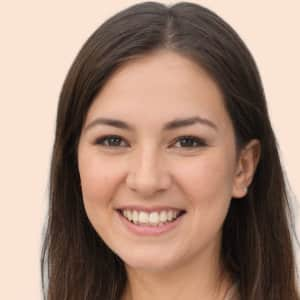## AQA: GCSE Maths Exam Papers

Download AQA GCSE Mathematics past exam papers to practice so that you prepare yourself for your final exam.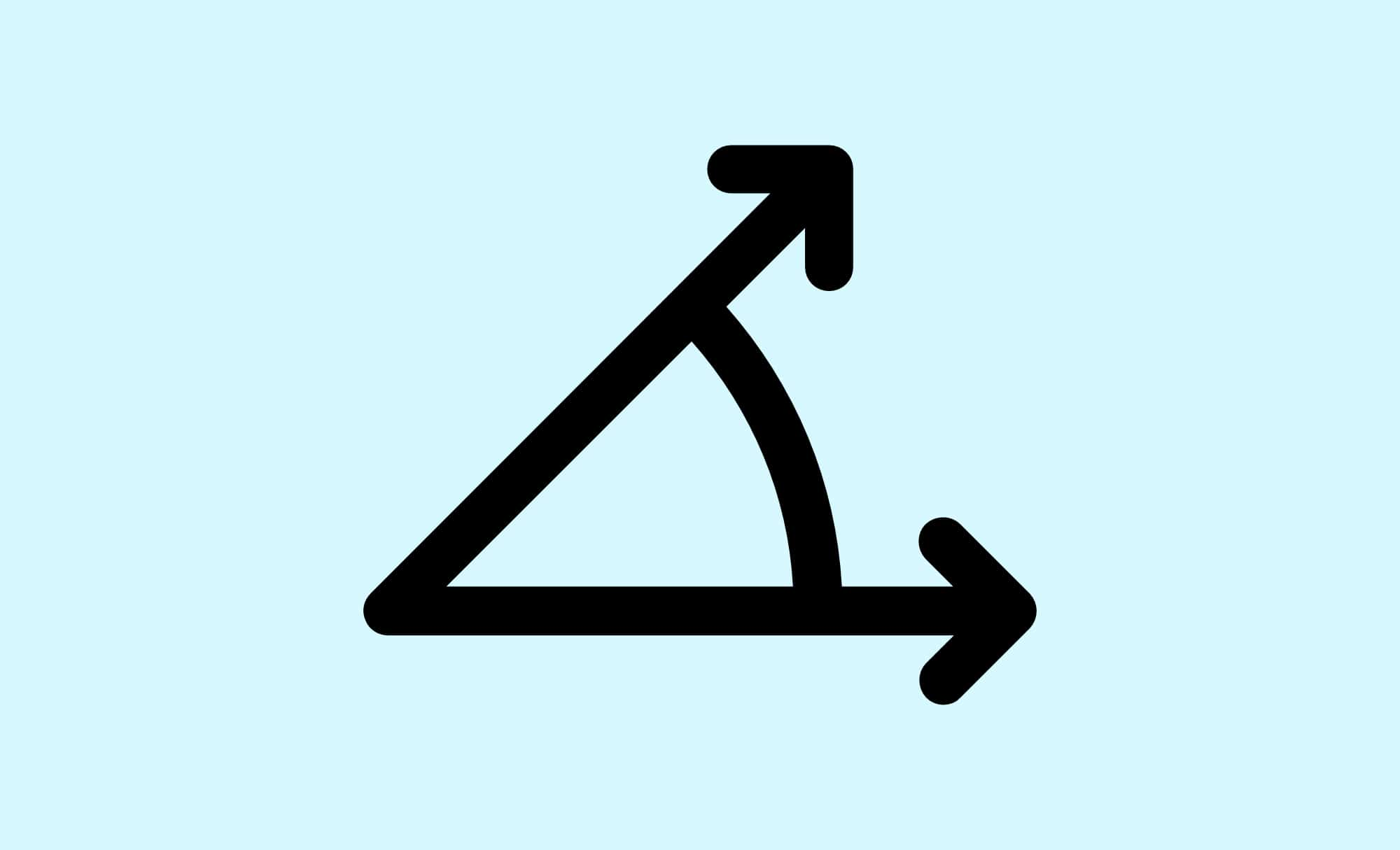## Angles Quiz P2

Angles are measured in degrees, written °. The maximum angle is 360°. This is the angle all the way around a point.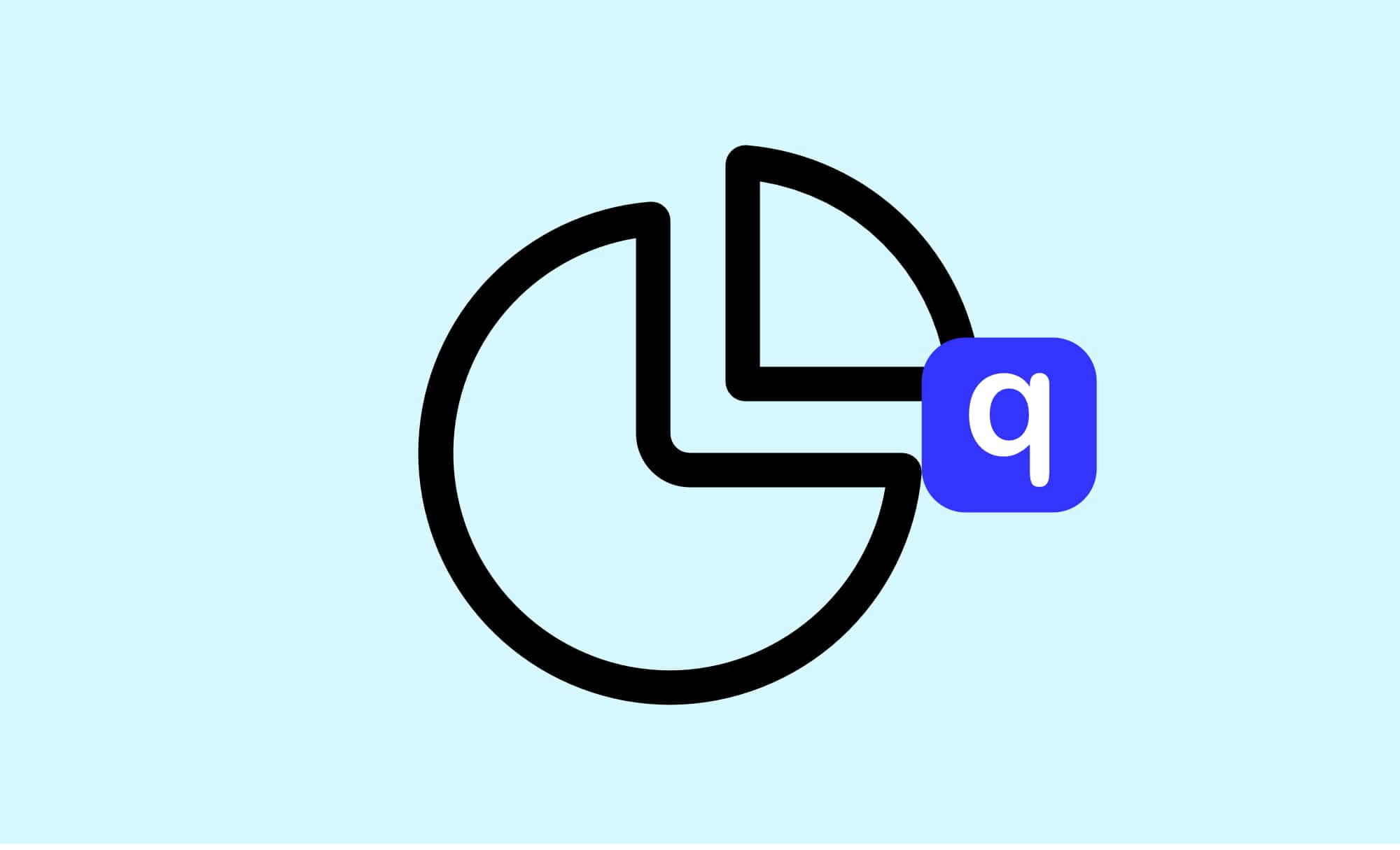## Fractions P1

Fractions are used commonly in everyday life, eg sale prices at 1/3 off, or recipes using 1/2 a tablespoon of an ingredient.## Linear Equations Word Problems P2

Linear equations are equations of the first order. These equations are defined for lines in the coordinate system.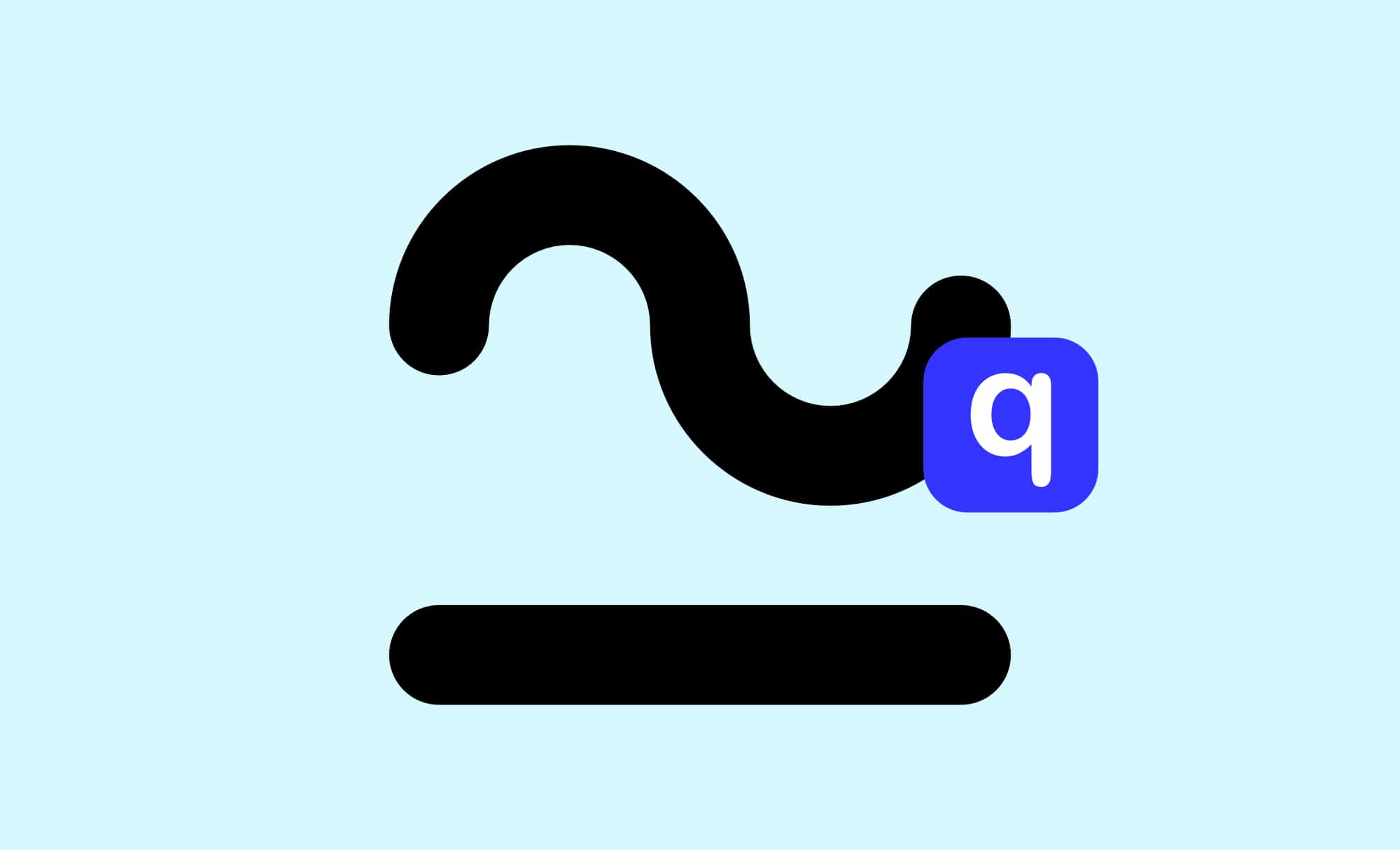## Congruence P1

Congruent shapes are identical but maybe reflected, rotated or translated. It is useful to know when triangles are Congruent.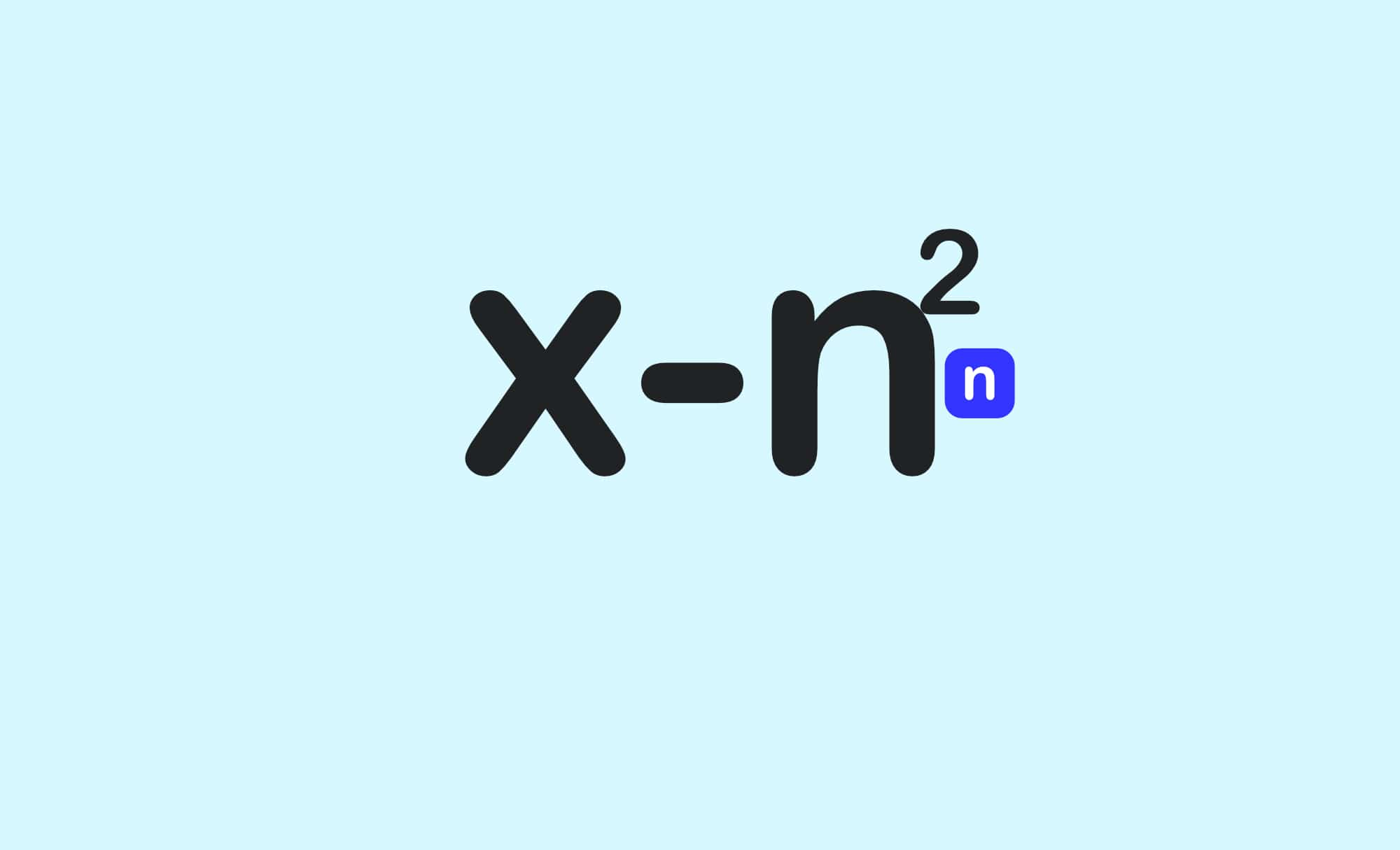## Multiplying and Dividing Algebra

In this section we are going to look at multiplying and dividing in algebra. Here are a few simple rules to help you get started with multiplying algebra. It is very similar to multiplying numbers.

You’ve successfully subscribed to GCSE.CO.UK
Welcome back! You’ve successfully signed in.
Great! You’ve successfully signed up.